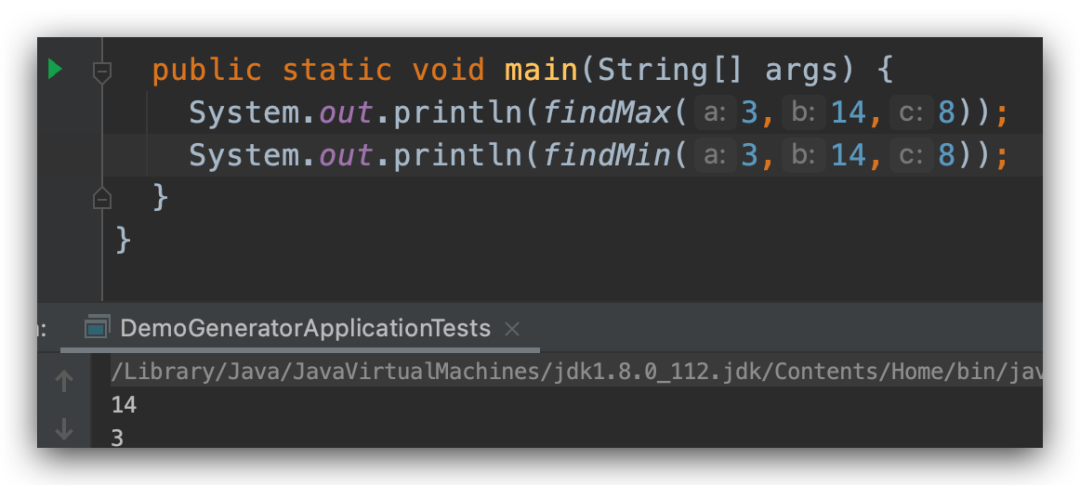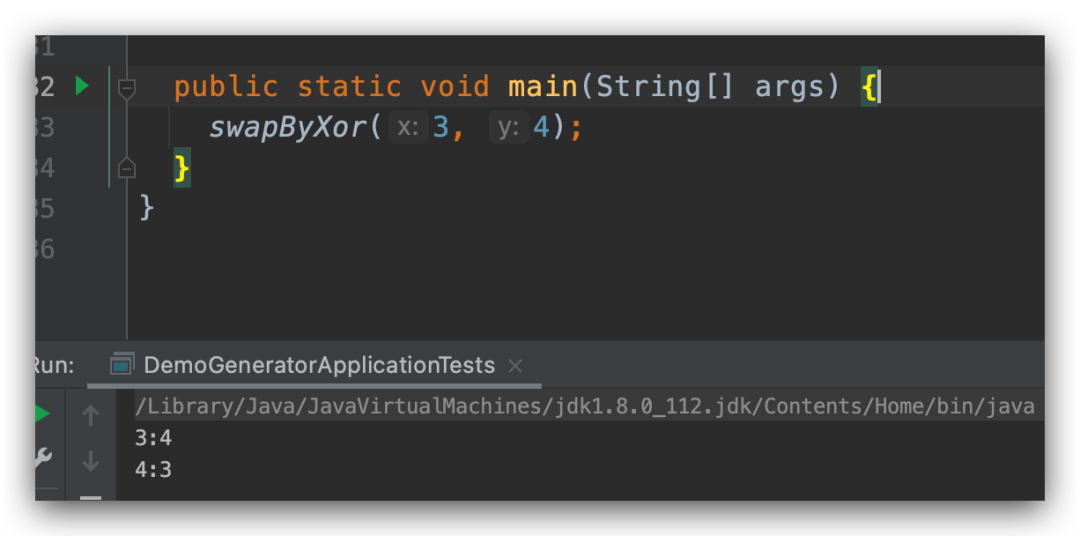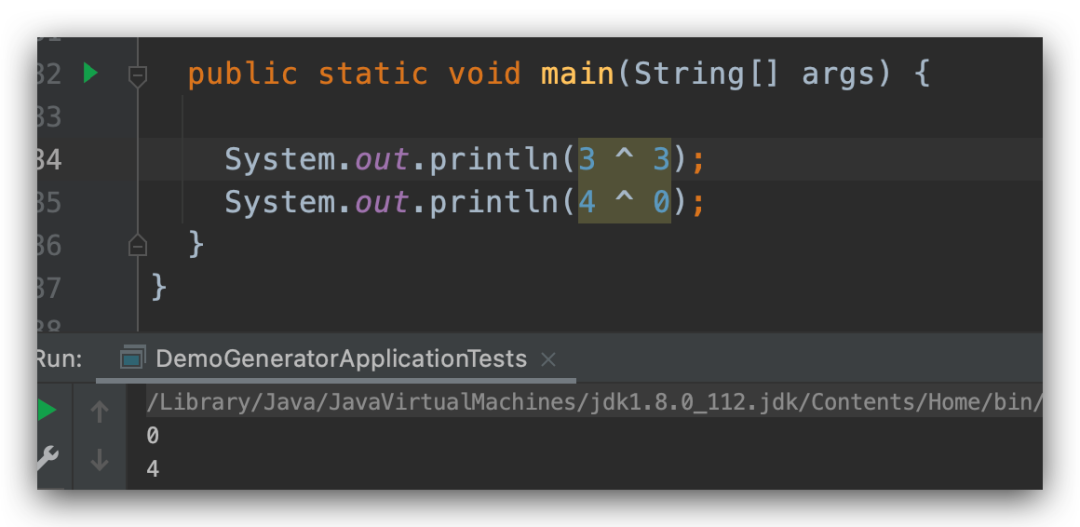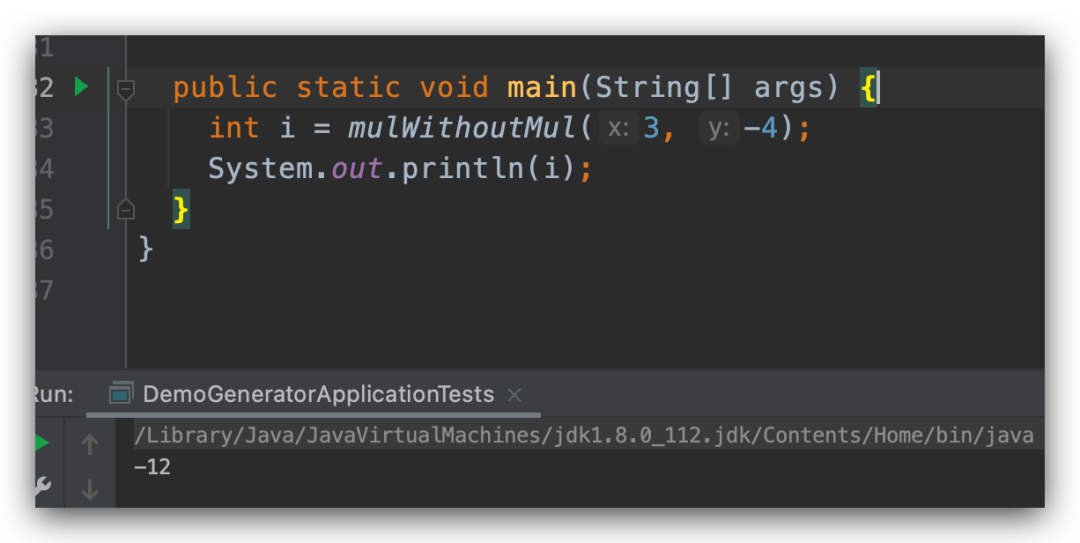# 教你几个 Java 中的奇技淫巧

nill0705

0收藏

1、找到最大值和最小值

``````public static int findMax(int a, int b, int c) {
int max = a;
boolean b1 = (max < b) && ((max = b) > 0);
b1 = (max < c) && ((max = c) > 0);
return max;
}

public static int findMin(int a, int b, int c) {
int min = a;
boolean b1 = (min > b) && ((min = b) > 0);
b1 = (min > c) && ((min = c) > 0);
return min;
}````````````public static int findPositiveMax(int a, int b, int c) {
int max = 0;
while (a > 0 || b > 0 || c > 0) {
a--;
b--;
c--;
max++;
}
return max;
}

public static int findPositiveMin(int a, int b, int c) {
int min = 0;
while (a > 0 && b > 0 && c > 0) {
a--;
b--;
c--;
min++;
}
return min;
}``````

2、不使用临时变量交换变量

swap(x, y) 操作大家都知道，就是交互 x 和 y 的值，比如 x = 3, y = 4; 在经过 swap 操作过后，x = 4，y = 3；我们这里的问题是如何在不使用临时变量的情况下，只有一行代码来实现这个方法。

``````public static void swapByXor(int x, int y) {
System.out.println(x + ":" + y);
x = x ^ y ^ (y = x);
System.out.println(x + ":" + y);
}````````````public static void swapByAddAndSub(int x, int y) {
System.out.println(x + ":" + y);
x = x + y - (y = x);
System.out.println(x + ":" + y);
}

public static void swapByMulAndDiv(int x, int y) {
System.out.println(x + ":" + y);
x = (x * y) / (y = x);
System.out.println(x + ":" + y);
}``````

3、两个数相乘

``````public static int mulWithoutMul(int x, int y) {
if (y == 0)
return0;
if (y > 0)
return (x + mulWithoutMul(x, y - 1));
return -mulWithoutMul(x, -y);
}``````4、不使用乘号计算乘以 15帖子
视频
声望
粉丝
相关问题
社区精华内容